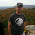VHDL coding tips and tricks: Not enough I/O pins in the FPGA board for your design?

## Saturday, April 28, 2012

### Not enough I/O pins in the FPGA board for your design?

Thanks to all the hardwork you have done, you successfully completed writing your first vhdl code. It did great in the functional simulation part. Now its time to test it on a FPGA board.

But looking at the board in hand, you realize that there are not enough input or output pins on the board to test the design. As you may have seen most of the FPGA boards have 8 switch inputs, 4 push buttons, 8 led's. There are other ways to increase I/O by using features like seven segment display, VGA monitor, RS232, DAC and ADC etc. But these features may make the design pretty complex and time consuming.

In such cases we can simply use the basic led's or switches in a multiplexed manner. In this article I have shown how to tackle such a problem in case you are out of input pins. But the same concept apply for output pins also.

Our design is named as my_design which has a 32 bit input and 8 bit output. Considering a typical FPGA board, we dont have 32 switches or push buttons. Suppose we have 8 switches. The idea is to apply the input in four stages of 8 bit each. This is how you do it.

1st stage : Set switches for 1st byte(LSB), press the push button.
2nd stage : change switches for 2nd byte and press the push button again.
3rd stage : change switches for 3rd byte, press the push button again.
4th stage : change switches for 4th byte(MSB) and press the push button again.

The code for my_design:

library IEEE;
use IEEE.STD_LOGIC_1164.ALL;

entity my_design is
Port ( input : in  STD_LOGIC_VECTOR (31 downto 0);
output : out  STD_LOGIC_VECTOR (7 downto 0));
end my_design;

architecture Behavioral of my_design is

begin

output <= input(31 downto 24) and input(23 downto 16)
and input(15 downto 8) and input(7 downto 0);

end Behavioral;

The code just does the AND operation between the 4 bytes of the 32 bit number entered.

Now the code for reducing the input numbers. I call this module as a wrapper module. This job of this module is to get the input in stages, concatenate it together and apply it to the instantiated my_design.

Code for wrapper module :

library IEEE;
use IEEE.STD_LOGIC_1164.ALL;
use IEEE.STD_LOGIC_UNSIGNED.ALL;
use IEEE.STD_LOGIC_ARITH.ALL;

entity wrapper is
Port ( Clk : in  STD_LOGIC;
push : in STD_LOGIC;
input : in  STD_LOGIC_VECTOR (7 downto 0);
output : out  STD_LOGIC_VECTOR (7 downto 0));
end wrapper;

architecture Behavioral of wrapper is

component my_design
port(   input : in  STD_LOGIC_VECTOR (31 downto 0);
output : out  STD_LOGIC_VECTOR (7 downto 0));
end component;

--state machine type
type stype is (idle,get_byte,delay);
signal s : stype := idle;
signal c1,c2 : integer := 0;
signal temp_reg : std_logic_vector(31 downto 0) := (others => '0');

begin

uut : my_design port map
(input => temp_reg, --concatenated signal
output => output    );

process(Clk)
begin
if(rising_edge(clk)) then
case s is
when idle =>
if(push = '1') then
s <= get_byte;
c1 <= c1+1;
end if;
when get_byte =>
temp_reg( (8*c1-1) downto (8*(c1-1)) ) <= input;
s <= delay;
when delay => --delay for a time gap.
--this delay is required to avoid the same byte getting
--registered into temp_reg for a single push button click.
c2 <= c2+ 1;
if(c2=25000000) then --for a 50 mhz clock, this generates a 0.5 sec delay.
c2 <= 0;
s <= idle;
if(c1=4) then
c1 <= 0;
end if;
end if;
end case;
end if;
end process;

end Behavioral;

I am using a state machine in the code, to get this done. The state machine has 3 stages.
1)idle - here system waits for a push button click.
2)get_byte - the system gets the switch inputs and stores in the temp_reg.
3)delay - system waits for a particular time(here 0.5 sec) doing nothing. This is to avoid duplicate registering of the same input.

A testbench was created for testing the design. The simulation waveforms are given below for your better understanding.

Note :- In a similar way you can also use multiplexed outputs.

#### 5 comments:

1.hello i have a doubt if my code as took IOBs higher amount of resources how we can reduce using synthesis tool settings

1.@ranga try using bram at the output...

2.Hey plz i want help for my project, plz help me,

3.please send the vhdl code for image edge detection based on FPGA using canny operator.
My email id is anphyjose18@gmail.com

4.Is there any reason that the delay state waits 0.5 seconds? If the user holds down the button for longer than that, wouldn't this logic read the data multiple times for one button press?

Wouldn't it make more sense to have the delay state wait until the button is released before returning to the idle state?